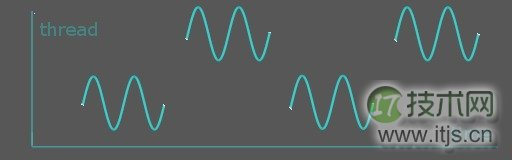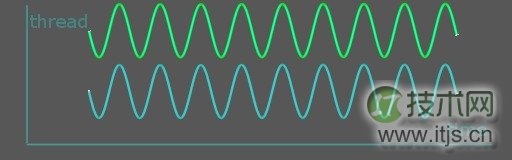﻿ 使用 C++ 11 编写 Linux 多线程程序_入门教程_IT技术网 ﻿

# 使用 C++ 11 编写 Linux 多线程程序

## 认识多线程

##### 图 1、单个 CPU 内核上的多个线程运行示意图##### 图 2、双核 CPU 上的多个线程运行示意图```namespace std{
// native_handle_type 是连接 thread 类和操作系统 SDK API 之间的桥梁。
typedef implementation-dependent native_handle_type;
native_handle_type native_handle();
//
struct id{
id() noexcept;
// 可以由==, < 两个运算衍生出其它大小关系运算。
template<class charT, class traits>
basic_ostream<charT, traits>&
// 哈希函数
template <class T> struct hash;
};
id get_id() const noexcept;
// 构造与析构
template<class F, class… Args> explicit thread(F&f, Args&&… args);
//
bool joinable() const noexcept;
void join();
void detach();
// 获取物理线程数目
static unsigned hardware_concurrency() noexcept;
}
void yield();
template<class Clock, class Duration>
void sleep_until(const chrono::time_point<Clock, Duration>& abs_time);
template<class Rep, class Period>
void sleep_for(const chromo::duration<Rep, Period>& rel_time);
}
}```

## 如何创建和结束一个线程

```int funcReturnInt(const char* fmt, ...){
va_list ap;
va_start(ap, fmt);
vprintf( fmt, ap );
va_end(ap);
return 0xabcd;
}
t->join();
delete t;
}

```void threadRunLambda(void){
int a = 100,
b = 200;
cout << (ia + ib) << endl;
},
a,
b );
t->join();
delete t;
}```

```struct God{
void create(const char* anything){
cout << "create " << anything << endl;
}
};
God god;
t->join();
delete t;
}```

```static void on_signal_term(int sig){
cout << "on SIGTERM:" << this_thread::get_id() << endl;
}
signal(SIGTERM, on_signal_term);
while(true){
++counter;
}
});
cout << "tid=" << tid << endl;
// 确保子线程已经在运行。
t->join();
delete t;
cout << "thread destroyed." << endl;
}```

```void threadMove(void){
int a = 1;
for(;;){
*pa = (*pa * 33) % 0x7fffffff;
if ( ( (*pa) >> 30) & 1) break;
}
}, &a);
thread t2 = move(t);	// 改为 t2 = t 将不能编译。
t2.join();
cout << "a=" << a << endl;
}```

```void threadSwap(void){
int a = 1;
for(;;){
*pa = (*pa * 33) % 0x7fffffff;
if ( ( (*pa) >> 30) & 1) break;
}
}, &a);
cout << "before swap: t=" << t.get_id()
<< ", t2=" << t2.get_id() << endl;
swap(t, t2);
cout << "after swap : t=" << t.get_id()
<< ", t2=" << t2.get_id() << endl;
t2.join();
cout << "a=" << a << endl;
}```

```void threadMoveTerm(void){
int a = 1;
for(;;){
*pa = (*pa * 33) % 0x7fffffff;
if ( ( (*pa) >> 30) & 1) break;
}
}, &a);
int i = 0;
for(;;)i++;
} );
t2 = move(t);	// 将会导致 std::terminate()
cout << "should not reach here" << endl;
t2.join();
}```

```class MyThread : public thread{
public:
template<typename Callable, typename... Args>
explicit
std::forward<Args>(args)...){
}
// disable copy constructors
};```

delete tp;

## 线程的调度

```void threadYield(void){
unsigned int procs = thread::hardware_concurrency(), // 获取物理线程数目
i = 0;
struct timeval t1, t2;
gettimeofday(&t1, NULL);
for(int i = 0, m = 13; i < COUNT; i++, m *= 17){
}
gettimeofday(&t2, NULL);
print_time(t1, t2, " with yield");
} );
for( i = 0; i < procs; i++){
struct timeval t1, t2;
gettimeofday(&t1, NULL);
for(int i = 0, m = 13; i < COUNT; i++, m *= 17){
do_nothing();
}
gettimeofday(&t2, NULL);
print_time(t1, t2, "without yield");
});
}
ta->join();
delete ta;
for( i = 0; i < procs; i++){
tb[i]->join();
delete tb[i];
};
delete tb;
}```

ta 线程因为需要经常切换去重新等待调度，它运行的时间要比 tb 要多，比如在作者的机器上运行得到如下结果：

```\$time ./a.out
without yield elapse 0.050199s
without yield elapse 0.051042s
without yield elapse 0.05139s
without yield elapse 0.048782s
with yield elapse 1.63366s
real	0m1.643s
user	0m1.175s
sys	0m0.611s```

ta 线程即使扣除系统调用运行时间 0.611s 之后，它的运行时间也远大于没有进行切换的线程。

## 线程间的数据交互和数据争用 (Data Racing)

```static void
inc(int *p ){
for(int i = 0; i < COUNT; i++){
(*p)++;
}
}
int a = 0;
ta.join();
tb.join();
cout << "a=" << a << endl;
}```

```static void
inc(atomic<int> *p ){
for(int i = 0; i < COUNT; i++){
(*p)++;
}
}
atomic<int> a(0) ;
ta.join();
tb.join();
cout << "a=" << a << endl;
}```

```static mutex g_mutex;
static void
inc(int *p ){
for(int i = 0; i < COUNT; i++){
lock_guard<mutex> _(g_mutex);
(*p)++;
}
}
int a = 0;
ta.join();
tb.join();
cout << "a=" << a << endl;
}```

```static mutex g_mutex;
static void
inc(int *p ){
thread_local int i; // TLS 变量
for(; i < COUNT; i++){
g_mutex.lock();
(*p)++;
g_mutex.unlock();
}
}
int a = 0;
ta.join();
tb.join();
cout << "a=" << a << endl;
}```

```#include <thread>
#include <iostream>
#include <condition_variable>
using namespace std;
mutex m;
condition_variable cv;
int i;
for(i = 0; i < THREAD_COUNT; i++){
t[i] = new thread( [](int index){
unique_lock<mutex> lck(m);
cv.wait_for(lck, chrono::hours(1000));
cout << index << endl;
}, i );
}
for(i = 0; i < THREAD_COUNT; i++){
lock_guard<mutex> _(m);
cv.notify_one();
}
for(i = 0; i < THREAD_COUNT; i++){
t[i]->join();
delete t[i];
}
delete t;
}```

## 几个高级概念

promise/future 可以用来在线程之间进行简单的数据交互，而不需要考虑锁的问题，线程 A 将数据保存在一个 promise 变量中，另外一个线程 B 可以通过这个 promise 变量的 get_future() 获取其值，当线程 A 尚未在 promise 变量中赋值时，线程 B 也可以等待这个 promise 变量的赋值：

```promise<string> val;
static void
future<string> fu = val.get_future();
cout << "waiting promise->future" << endl;
cout << fu.get() << endl;
});
val.set_value("promise is set");
});
ta.join();
tb.join();
}```

```static mutex g_mutex;
static void
auto run = [=](int index){
{
lock_guard<mutex> _(g_mutex);
cout << "tasklet " << index << endl;
}
return index * 1000;
};
int f1 = pt1.get_future().get();
int f2 = pt2.get_future().get();
cout << "task result=" << f1 << endl;
cout << "task result=" << f2 << endl;
t1.join();
t2.join();
}```

```static long
do_sum(vector<long> *arr, size_t start, size_t count){
static mutex _m;
long sum = 0;
for(size_t i = 0; i < count; i++){
sum += (*arr)[start + i];
}
{
lock_guard<mutex> _(_m);
<< ", count=" << count
<< ", sum=" << sum << endl;
}
return sum;
}
static void
# define COUNT 1000000
vector<long> data(COUNT);
for(size_t i = 0; i < COUNT; i++){
data[i] = random() & 0xff;
}
//
vector< future<long> > result;
size_t ptc = thread::hardware_concurrency() * 2;
for(size_t batch = 0; batch < ptc; batch++){
size_t batch_each = COUNT / ptc;
if (batch == ptc - 1){
batch_each = COUNT - (COUNT / ptc * batch);
}
result.push_back(async(do_sum, &data, batch * batch_each, batch_each));
}
long total = 0;
for(size_t batch = 0; batch < ptc; batch++){
total += result[batch].get();
}
cout << "total=" << total << endl;
}```

## 几个需要注意的地方

```static mutex g_mutex1, g_mutex2;
static void
inc1(int *p ){
for(int i = 0; i < COUNT; i++){
g_mutex1.lock();
(*p)++;
g_mutex2.lock();
// do something.
g_mutex2.unlock();
g_mutex1.unlock();
}
}
static void
inc2(int *p ){
for(int i = 0; i < COUNT; i++){
g_mutex2.lock();
g_mutex1.lock();
(*p)++;
g_mutex1.unlock();
// do other thing.
g_mutex2.unlock();
}
}
int a = 0;
ta.join();
tb.join();
cout << "a=" << a << endl;
}```

```static mutex g_mutex1, g_mutex2;
static voi
inc1(int *p ){
for(int i = 0; i < COUNT; i++){
g_mutex1.lock();
(*p)++;
g_mutex1.unlock();
g_mutex2.lock();
// do something.
g_mutex2.unlock();
}
}
static void
inc2(int *p ){
for(int i = 0; i < COUNT; i++){
g_mutex2.lock();
// do other thing.
g_mutex2.unlock();
g_mutex1.lock();
(*p)++;
g_mutex1.unlock();
}
}
int a = 0;
ta.join();
tb.join();
cout << "a=" << a << endl;
}```

﻿